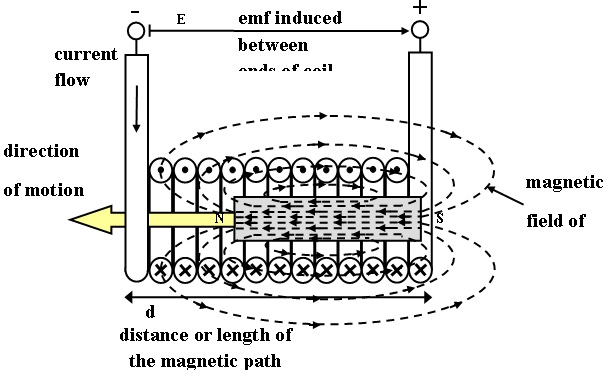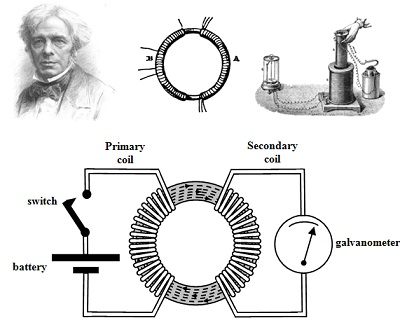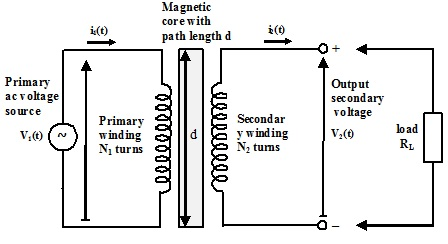#### Theory of Transformers and Faraday’s Experiments

EMF Induced in a Coil:

The principle of electromagnetic induction can be lengthened to the coil of several turns. Figure below shows a coil of N turns with a permanent magnet inserted within the coil. The direction of the emf is harder to establish here as the geometry doesn’t quite suit Fleming’s Right Hand Rule. Lenz’s Law is more obliging in determining the direction of the current flow. This will be such that it generates a magnetic field in the coil that opposes that of the magnet. In actuality there is, of course, interaction among such two fields, even although only that related with the magnet is shown in the diagram.Figure: Emf Induced in a Coil

When the total flux produced by the magnet is ? Webers and the magnet is moving with a velocity u, then the emf induced in single turn of the coil of length, l is given as:

e(t) = B l u = dΦ/dt V

If, though, the flux of the magnet cuts all the turns of the coil concurrently, then this emf is induced into all the turns of coil with cumulative effect and hence the total emf induced in a coil containing N turns is as follows:

Induced emf, e(t) = N (dΦ/dt) V

This result is termed as the Faraday’s Law.

In the year1831 Michael Faraday (1791-1867), a British physicist/chemist frequently hailed in England as the father of Electrical Engineering, taken out a number of experiments which are usually accepted as the discovery of electromagnetic induction. One of such is reproduced in figure below along with several other illustrations.Figure: Faraday’s Experiment on Electromagnetic Induction

In the experiment depicted, two coils are wound around a magnetic core. The primary coil is joined to a dc battery through a switch whereas the secondary coil is joined to a Galvanometer that is a sensitive meter for measuring the current. Faraday observed that whenever he closed the switch, the indicator on Galvanometer in brief registered a current that quickly decayed to zero. He then observed that whenever he opened the switch again, the Galvanometer in brief registered a current in the reverse direction of similar magnitude that again quickly decayed to zero. This was repeated as often and as quickly as he opened and closed the switch.

What happens is that when the switch is closed, the current flowing in the primary coil induces the magnetic flux in core. While this is being established the flux is modifying. The changing flux cuts the secondary coil and induces an emf in this coil that causes current to flow via the Galvanometer. Once the magnetic flux has stabilised, though, its rate of change drops to zero and therefore as well does the emf and current induced in the secondary coil. Whenever the switch is opened current ceases flowing in the primary coil and therefore the flux in the core decays to zero. While this flux is changing again, it induces an emf in the secondary coil in the opposite direction, which then vanishes whenever the flux has decayed to zero.

This experiment joins the effects of magnetomotive force produced in the core through current flowing in the primary coil and the emf induced in the secondary coil by the magnetic flux changing in the core. This proves that electrical energy can be transferred from one circuit to other through the medium of magnetism. This principle is exploited in the electrical transformers.

It is as well significant, though, to interpret Lenz’s Law at this point to its full degree. This points out that whenever a current flow in the primary coil creating a magnetic flux and inducing an emf in the secondary coil, there is as well an opposing emf produced in the primary coil itself that tends to oppose the flow of current and the resultant flux produced. This is termed to as a Back-emf and gives a formal statement of the Lenz’s Law as:

Back emf, eb(t) = -N(dΦ/dt) V

The Ideal Transformer:

In Faraday’s experiment, the Galvanometer registers a current only for a short period of time whereas the magnetic flux is changing. Whenever the flux is constant or zero, its rate of change is zero and no emf is induced in the secondary coil beneath such conditions.

Whenever the source driving the primary coil is made an ac sinusoidal source, then the current in primary coil will be constantly changing. This in turn makes sure that the flux in the core is changing constantly and thus that there is a continuous emf induced in secondary coil. This is the principle of operation of transformer an electrical representation of which is shown in figure below.Figure: Electrical Representation of a Transformer

The operation is as shown. The ac voltage source applied to primary winding, V1(t) causes a current to flow in the primary coil, i1(t). This current then forms a magnetic flux, Φ in the core of transformer on which both primary and secondary coils are wound. The flux then links the secondary coil and induces an emf in it that is measured across the output terminals as the voltage, V2(t). When a load is joined to the output of the transformer a current, i2(t) will flow in it, delivering power to load.

When the primary winding is driven with a voltage, V1(t) and the resultant current flowing in this winding is i1(t), then when the primary coil has N1 turns this will make a flux in the core given as:

Φ(t) = N1i1(t)

From Lenz’s Law, the back-emf produced by this flux will be equivalent to the source voltage and is given as:

e1(t) = -N1(dΦ/dt) = V1(t)

Thus,

dΦ/dt = V1(t)/N1

For an ideal transformer it is supposed that all the flux produced in the core by primary winding really cuts the secondary winding also, that is, there is no leakage of flux. When this is the case then the emf induced in secondary winding that has N2 turns is as follows:

e2(t) = -N2(dΦ/dt) = V2(t)

And hence,

dΦ/dt = V2(t)/N2

As the flux is identical cutting both primary and secondary coils, the two expressions can be equated to provide:

V2(t)/N2 = V1(t)/N1

Or,

V2(t) = (N2/N1) V1(t)

When the source voltage is provided as:

V1(t) = V1m sin ωt

Then the secondary output voltage is provided by:

V2(t) = (N2/N1) V1m sin ωt

And hence,

V2(t) = (N2/N1) V1m sin (ωt) = V2m sin (ωt)

From this we can state the basic property of the transformer:

Turn Ratio = n = V2m/V1m = N2/N1

The above relationship exhibits that the magnitude of output voltage of the secondary winding is scaled by turn ratio, n, of the transformer. This can be higher than or less than unity therefore the magnitude of the secondary output voltage can be made higher, termed to as step-up, or lower, termed to as step-down, than the primary input voltage. The phase of secondary voltage can be set up to be inverted with respect to that of primary voltage by modifying the relative directions of the primary and secondary windings. This can as well be accomplished by modifying the relative connections to the primary and secondary windings as they are however isolated from one other. Furthermore, by taking a centre-tap connection off the secondary winding equivalent anti-phase output voltages can be received. This is helpful in generating matched negative and positive secondary voltages for use in a dual polarity power supply, for instance.

As the turns ratio is a fixed physical property of transformer, the ratio applies to instantaneous, peak or rms values of primary and secondary voltages and hence:

Turns ration = n = N2/N1 = V2(t)/V1(t) = V2m/V1m = V2rms/V1rms

Illustration: A step-down transformer is employed to decrease the mains voltage from 220 Vrms to the peak secondary voltage of 18Vpk. When the primary winding has 5000 turns then find out the number of turns required on the secondary winding.

Solution:

When the secondary voltage is 18V pk then the rms value is:

V2rms = 18/√2 = 18 x 0.707 = 12.726 V

Then,

Turn ration = n = N2/N1 = V2rms/ V1rms = 12.726/220 = 0.058

And hence,

N2= n x N1 = 0.058 x 5000 = 290 turns

Current Ratio:

The main aim of voltage transformers is to permit power to be delivered to a load at a distinct voltage to that at which it is produced or distributed, as for illustration in the case of distribution of mains domestic electricity. In an ideal transformer there is no loss of power and hence all power fed into the primary input of the transformer must be delivered to the load joined to the output of the secondary winding. When average power is formed the focus then:

P2AVE = P1AVE

And hence,

V2rms I2rms = V1rms I1rms

I2rms/I1rms = V1rms/V2rms =1/n

This exhibits that the ratio of currents in a transformer is the inverse of the ratio of corresponding voltages.

Illustration:  A transformer fed from the 220V mains supply should deliver 0.55kW of power to a motor that draws a rated current of 10 A rms. The transformer should contain a total number of turns of 10,000. Find out:

a) The rated voltage of motor
b) The number of turns needed on each and every winding of the transformer
c) The current which should be supplied to the transformer.

Solution:

The power that should be delivered to the load is P2AVE = 0.55 kW = 550 W
Then:

a) V2rms I2rms = 550 W and I2rms = 10 A and hence, V2rms = 550/10 = 55 V rms

b) V1rms = 220 V then, n = V2rms/I2rms = 55/220 = 0.25

n = N2/N1 = 0.25

N2 = 0.25 N1

Total turns is N1 + N2 = N1 + 0.25 N1 = 1.25 N1 = 10,000

Then, N1 = 10,000/1.25 = 8,000 and N2 = 0.25 N1 = 0.25 x 8,000 = 2,000

c) I2rms/ I1rms = 1/n and hence, I1rms = n I2rms = 0.25 x 10 = 2.5 A rms

Latest technology based Electrical Engineering Online Tutoring Assistance

Tutors, at the www.tutorsglobe.com, take pledge to provide full satisfaction and assurance in Electrical Engineering help via online tutoring. Students are getting 100% satisfaction by online tutors across the globe. Here you can get homework help for Electrical Engineering, project ideas and tutorials. We provide email based Electrical Engineering help. You can join us to ask queries 24x7 with live, experienced and qualified online tutors specialized in Electrical Engineering. Through Online Tutoring, you would be able to complete your homework or assignments at your home. Tutors at the TutorsGlobe are committed to provide the best quality online tutoring assistance for Electrical Engineering Homework help and assignment help services. They use their experience, as they have solved thousands of the Electrical Engineering assignments, which may help you to solve your complex issues of Electrical Engineering. TutorsGlobe assure for the best quality compliance to your homework. Compromise with quality is not in our dictionary. If we feel that we are not able to provide the homework help as per the deadline or given instruction by the student, we refund the money of the student without any delay.